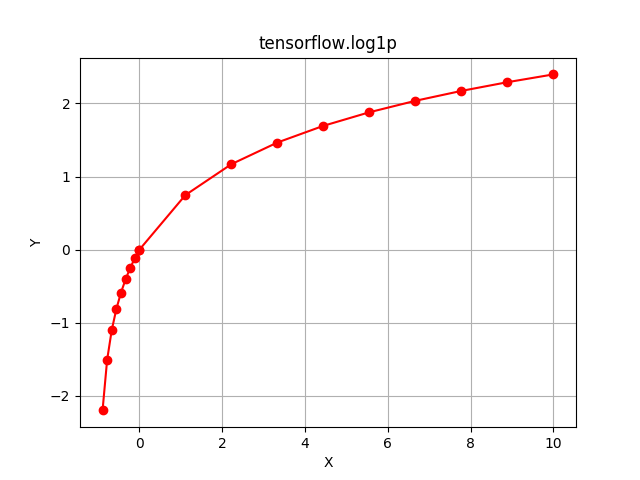GeeksforGeeks App
Open AppBrowser
Continue

# Python | Tensorflow log1p() method

Tensorflow is an open-source machine learning library developed by Google. One of its applications is to develop deep neural networks.
The module tensorflow.math provides support for many basic mathematical operations. Function tf.log1p() [alias tf.math.log1p] provides support for the natural logarithmic function in Tensorflow. It expects the input in form of complex numbers asor floating point numbers. The input type is tensor and if the input contains more than one element, an element-wise logarithm ofis computed,.

Syntax: tf.log1p(x, name=None) or tf.math.log1p(x, name=None)
Parameters
x: A Tensor of type bfloat16, half, float32, float64, complex64 or complex128.
name (optional): The name for the operation.
Return type: A Tensor with the same size and type as that of x.

Code #1:

## Python3

 `# Importing the Tensorflow library``import` `tensorflow as tf` `# A constant vector of size 5``a ``=` `tf.constant([``-``1.5``, ``-``1``, ``-``0.5``, ``0``, ``0.5``, ``1``, ``1.5``], dtype ``=` `tf.float32)` `# Applying the log1p function and``# storing the result in 'b'``b ``=` `tf.log1p(a, name ``=``'log1p'``)` `# Initiating a Tensorflow session``with tf.Session() as sess:``    ``print``(``'Input type:'``, a)``    ``print``(``'Input:'``, sess.run(a))``    ``print``(``'Return type:'``, b)``    ``print``(``'Output:'``, sess.run(b))`

Output:

```Input type: Tensor("Const:0", shape=(7, ), dtype=float32)
Input: [-1.5 -1.  -0.5  0.   0.5  1.   1.5]
Return type: Tensor("log1p:0", shape=(7, ), dtype=float32)
Output: [        nan        -inf -0.6931472   0.          0.4054651   0.6931472
0.91629076]```denotes that natural logarithm of 1+x doesn’t exist for negative values anddenotes that it approaches to negative infinity as the input approaches to -1.
Code #2: Visualization

## Python3

 `# Importing the Tensorflow library``import` `tensorflow as tf` `# Importing the NumPy library``import` `numpy as np` `# Importing the matplotlib.pyplot function``import` `matplotlib.pyplot as plt` `# A vector of size 20 with values from -1 to 0 and 0 to 10``a ``=` `np.append(np.linspace(``-``1``, ``0``, ``10``), np.linspace(``0``, ``10``, ``10``))` `# Applying the logarithmic function and``# storing the result in 'b'``b ``=` `tf.log1p(a, name ``=``'log1p'``)` `# Initiating a Tensorflow session``with tf.Session() as sess:``    ``print``(``'Input:'``, a)``    ``print``(``'Output:'``, sess.run(b))``    ``plt.plot(a, sess.run(b), color ``=` `'red'``, marker ``=` `"o"``)``    ``plt.title(``"tensorflow.abs"``)``    ``plt.xlabel(``"X"``)``    ``plt.ylabel(``"Y"``)``    ``plt.grid()` `    ``plt.show()`

Output:

```Input: [-1.         -0.88888889 -0.77777778 -0.66666667 -0.55555556 -0.44444444
-0.33333333 -0.22222222 -0.11111111  0.          0.          1.11111111
2.22222222  3.33333333  4.44444444  5.55555556  6.66666667  7.77777778
8.88888889 10.        ]
Output: [       -inf -2.19722458 -1.5040774  -1.09861229 -0.81093022 -0.58778666
-0.40546511 -0.25131443 -0.11778304  0.          0.          0.7472144
1.17007125  1.46633707  1.69459572  1.88031287  2.03688193  2.17222328
2.29141179  2.39789527]```My Personal Notes arrow_drop_up